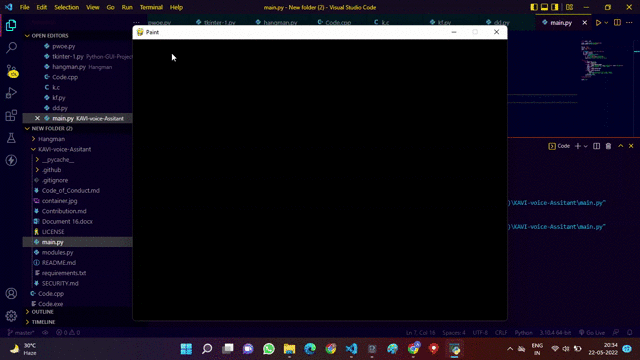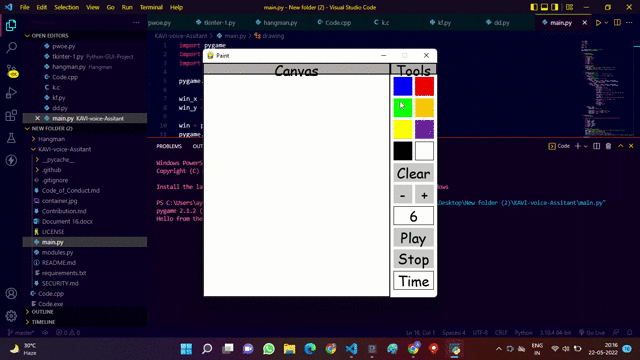# How to create MS Paint clone with Python and PyGame?

• Last Updated : 01 Jul, 2022

In this article, we will create a simple MS paint program with Python and PyGame.

MS Paint is a simple program made by Microsoft, it allows users to create basic art and painting. Since its inception, MS Paint has been included with every version of Microsoft Windows. MS Paint provides features for drawing and painting in color and tools to create geometrical designs. In this section, we will try to make a simple copy of an MS paint with the help of a python module such as Pygame, random, and os. these modules will help us to make our simple canvas. Before moving further we will discuss some basic concepts of these modules:

• Pygame:  It consists of computer graphics. Pygame is an open-source set of Python Modules. And as the name suggests, it can be used to create games You can code the games and then use specific commands to change them into an executable file that you can share with your friends to show them the work you have been doing.  It includes  graphics and sound libraries designed for use with the Python programming language.
• os: This module is used to interact with the operating system.  This is a pre-installed module. The OS module in Python provides functions for interacting with the operating system. OS comes under Python’s standard utility modules.
• random: This module is used to generate random numbers. This is a pre-installed module. Python Random module is an in-built module of Python which is used to generate random numbers. These are pseudo-random numbers means these are not truly random. This module can be used to perform random actions such as generating random numbers, printing random a value for a list or string, etc.

Packages required

`pip install pygame`

### Example 1: Making A simple Paint Application Using Python

In this example, we will create a canvas in which we can draw a colorful line whenever we press down our mouse key. Every time when we release the mouse click it will choose a different random color with the help of a random module. if the user double clicks then the color of the brush is changed.
In the code, the round function takes an argument of screen size, the color of the brush, the Start and end position of the axis, and the radius of the brush. with the help of these arguments, we will draw a colorful line.

## Python3

 `import` `pygame``import` `random` `# Making canvas``screen ``=` `pygame.display.set_mode((``900``, ``700``))` `# Setting Title``pygame.display.set_caption(``'GFG Paint'``)`  `draw_on ``=` `False``last_pos ``=` `(``0``, ``0``)` `# Radius of the Brush``radius ``=` `5`  `def` `roundline(canvas, color, start, end, radius``=``1``):``    ``Xaxis ``=` `end[``0``]``-``start[``0``]``    ``Yaxis ``=` `end[``1``]``-``start[``1``]``    ``dist ``=` `max``(``abs``(Xaxis), ``abs``(Yaxis))``    ``for` `i ``in` `range``(dist):``        ``x ``=` `int``(start[``0``]``+``float``(i)``/``dist``*``Xaxis)``        ``y ``=` `int``(start[``1``]``+``float``(i)``/``dist``*``Yaxis)``        ``pygame.draw.circle(canvas, color, (x, y), radius)`  `try``:``    ``while` `True``:``        ``e ``=` `pygame.event.wait()``        ` `        ``if` `e.``type` `=``=` `pygame.QUIT:``            ``raise` `StopIteration``            ` `        ``if` `e.``type` `=``=` `pygame.MOUSEBUTTONDOWN:         ``            ``# Selecting random Color Code``            ``color ``=` `(random.randrange(``256``), random.randrange(``                ``256``), random.randrange(``256``))``            ``# Draw a single circle wheneven mouse is clicked down.``            ``pygame.draw.circle(screen, color, e.pos, radius)``            ``draw_on ``=` `True``        ``# When mouse button released it will stop drawing   ``        ``if` `e.``type` `=``=` `pygame.MOUSEBUTTONUP:``            ``draw_on ``=` `False``        ``# It will draw a continuous circle with the help of roundline function.   ``        ``if` `e.``type` `=``=` `pygame.MOUSEMOTION:``            ``if` `draw_on:``                ``pygame.draw.circle(screen, color, e.pos, radius)``                ``roundline(screen, color, e.pos, last_pos,  radius)``            ``last_pos ``=` `e.pos``        ``pygame.display.flip()` `except` `StopIteration:``    ``pass``  ` `# Quit``pygame.quit()`

Output:### Example 2: Making a paint application to draw and erase in Canvas

In this example, We made a canvas to draw with eight different colors to paint. It also includes features like a clear button which will clear the screen and give us a new canvas to draw, and a + and – button which increases thor decrease the brush size. Additionally, it also includes a clock timer to practice painting at a given interval of time.

## Python3

 `# import library``import` `pygame ``import` `random` `pygame.init()` `# Setting window size``win_x ``=` `500``win_y ``=` `500` `win ``=` `pygame.display.set_mode((win_x, win_y))``pygame.display.set_caption(``'Paint'``)` `# Class for drawing``class` `drawing(``object``):` `    ``def` `__init__(``self``):``        ``'''constructor'''``        ``self``.color ``=` `(``0``, ``0``, ``0``)``        ``self``.width ``=` `10``        ``self``.height ``=` `10``        ``self``.rad ``=` `6``        ``self``.tick ``=` `0``        ``self``.time ``=` `0``        ``self``.play ``=` `False``        ` `    ``# Drawing Function``    ``def` `draw(``self``, win, pos):``        ``pygame.draw.circle(win, ``self``.color, (pos[``0``], pos[``1``]), ``self``.rad)``        ``if` `self``.color ``=``=` `(``255``, ``255``, ``255``):``            ``pygame.draw.circle(win, ``self``.color, (pos[``0``], pos[``1``]), ``20``)` `    ``# detecting clicks``    ``def` `click(``self``, win, ``list``, list2):``        ``pos ``=` `pygame.mouse.get_pos()  ``# Localização do mouse` `        ``if` `pygame.mouse.get_pressed() ``=``=` `(``1``, ``0``, ``0``) ``and` `pos[``0``] < ``400``:``            ``if` `pos[``1``] > ``25``:``                ``self``.draw(win, pos)``        ``elif` `pygame.mouse.get_pressed() ``=``=` `(``1``, ``0``, ``0``):``            ``for` `button ``in` `list``:``                ``if` `pos[``0``] > button.x ``and` `pos[``0``] < button.x ``+` `button.width:``                    ``if` `pos[``1``] > button.y ``and` `pos[``1``] < button.y ``+` `button.height:``                        ``self``.color ``=` `button.color2``            ``for` `button ``in` `list2:``                ``if` `pos[``0``] > button.x ``and` `pos[``0``] < button.x ``+` `button.width:``                    ``if` `pos[``1``] > button.y ``and` `pos[``1``] < button.y ``+` `button.height:``                        ``if` `self``.tick ``=``=` `0``:``                            ``if` `button.action ``=``=` `1``:``                                ``win.fill((``255``, ``255``, ``255``))``                                ``self``.tick ``+``=` `1``                            ``if` `button.action ``=``=` `2` `and` `self``.rad > ``4``:``                                ``self``.rad ``-``=` `1``                                ``self``.tick ``+``=` `1``                                ``pygame.draw.rect(``                                    ``win, (``255``, ``255``, ``255``), (``410``, ``308``, ``80``, ``35``))` `                            ``if` `button.action ``=``=` `3` `and` `self``.rad < ``20``:``                                ``self``.rad ``+``=` `1``                                ``self``.tick ``+``=` `1``                                ``pygame.draw.rect(``                                    ``win, (``255``, ``255``, ``255``), (``410``, ``308``, ``80``, ``35``))` `                            ``if` `button.action ``=``=` `5` `and` `self``.play ``=``=` `False``:``                                ``self``.play ``=` `True``                                ``game()``                                ``self``.time ``+``=` `1``                            ``if` `button.action ``=``=` `6``:``                                ``self``.play ``=` `False``                                ``self``.time ``=` `0` `        ``for` `button ``in` `list2:``            ``if` `button.action ``=``=` `4``:``                ``button.text ``=` `str``(``self``.rad)` `            ``if` `button.action ``=``=` `7` `and` `self``.play ``=``=` `True``:``                ``button.text ``=` `str``(``40` `-` `(player1.time ``/``/` `100``))``            ``if` `button.action ``=``=` `7` `and` `self``.play ``=``=` `False``:``                ``button.text ``=` `'Time'` `# Class for buttons``class` `button(``object``):` `    ``def` `__init__(``self``, x, y, width, height, color, color2, outline``=``0``, action``=``0``, text``=``''):``        ``self``.x ``=` `x``        ``self``.y ``=` `y``        ``self``.height ``=` `height``        ``self``.width ``=` `width``        ``self``.color ``=` `color``        ``self``.outline ``=` `outline``        ``self``.color2 ``=` `color2``        ``self``.action ``=` `action``        ``self``.text ``=` `text``        ` `# Class for drawing buttons``    ``def` `draw(``self``, win):` `        ``pygame.draw.rect(win, ``self``.color, (``self``.x, ``self``.y,``                                           ``self``.width, ``self``.height), ``self``.outline)``        ``font ``=` `pygame.font.SysFont(``'comicsans'``, ``30``)``        ``text ``=` `font.render(``self``.text, ``1``, ``self``.color2)``        ``pygame.draw.rect(win, (``255``, ``255``, ``255``), (``410``, ``446``, ``80``, ``35``))``        ``# pygame.draw.rect(win, (255, 255, 255), (410, 308, 80, 35))``        ``win.blit(text, (``int``(``self``.x``+``self``.width``/``2``-``text.get_width()``/``2``),``                        ``int``(``self``.y``+``self``.height``/``2``-``text.get_height()``/``2``)))`  `def` `drawHeader(win):``    ``# Drawing header space``    ``pygame.draw.rect(win, (``175``, ``171``, ``171``), (``0``, ``0``, ``500``, ``25``))``    ``pygame.draw.rect(win, (``0``, ``0``, ``0``), (``0``, ``0``, ``400``, ``25``), ``2``)``    ``pygame.draw.rect(win, (``0``, ``0``, ``0``), (``400``, ``0``, ``100``, ``25``), ``2``)` `    ``# Printing header``    ``font ``=` `pygame.font.SysFont(``'comicsans'``, ``30``)` `    ``canvasText ``=` `font.render(``'Canvas'``, ``1``, (``0``, ``0``, ``0``))``    ``win.blit(canvasText, (``int``(``200` `-` `canvasText.get_width() ``/` `2``),``                          ``int``(``26` `/` `2` `-` `canvasText.get_height() ``/` `2``) ``+` `2``))` `    ``toolsText ``=` `font.render(``'Tools'``, ``1``, (``0``, ``0``, ``0``))``    ``win.blit(toolsText, (``int``(``450` `-` `toolsText.get_width() ``/` `2``),``                         ``int``(``26` `/` `2` `-` `toolsText.get_height() ``/` `2` `+` `2``)))`  `def` `draw(win):``    ``player1.click(win, Buttons_color, Buttons_other)` `    ``pygame.draw.rect(win, (``0``, ``0``, ``0``), (``400``, ``0``, ``100``, ``500``),``                     ``2``)  ``# Drawing button space``    ``pygame.draw.rect(win, (``255``, ``255``, ``255``), (``400``, ``0``, ``100``, ``500``),)``    ``pygame.draw.rect(win, (``0``, ``0``, ``0``), (``0``, ``0``, ``400``, ``500``),``                     ``2``)  ``# Drawing canvas space``    ``drawHeader(win)` `    ``for` `button ``in` `Buttons_color:``        ``button.draw(win)` `    ``for` `button ``in` `Buttons_other:``        ``button.draw(win)` `    ``pygame.display.update()`  `def` `main_loop():``    ``run ``=` `True``    ``while` `run:``        ``keys ``=` `pygame.key.get_pressed() ``        ``for` `event ``in` `pygame.event.get():``            ``if` `event.``type` `=``=` `pygame.QUIT ``or` `keys[pygame.K_ESCAPE]:``                ``run ``=` `False` `        ``draw(win)` `        ``if` `0` `< player1.tick < ``40``:``            ``player1.tick ``+``=` `1``        ``else``:``            ``player1.tick ``=` `0` `        ``if` `0` `< player1.time < ``4001``:``            ``player1.time ``+``=` `1``        ``elif` `4000` `< player1.time < ``4004``:``            ``gameOver()``            ``player1.time ``=` `4009``        ``else``:``            ``player1.time ``=` `0``            ``player1.play ``=` `False` `    ``pygame.quit()`  `def` `game():``    ``object` `=` `[``'Casa'``, ``'cachoro'``, ``'caneta'``, ``'bola de futebol'``, ``'caneca'``, ``'Computador'``,``              ``'Chocolate'``, ``'Jesus'``, ``'Celular'``, ``'Iphone'``, ``'Teclado(instrumento)'``, ``'teclado(computador)'``]` `    ``font ``=` `pygame.font.SysFont(``'comicsans'``, ``40``)``    ``font2 ``=` `pygame.font.SysFont(``'comicsans'``, ``25``)``    ``text ``=` `font.render(``'Sua Palavra é: '` `+``                       ``object``[random.randint(``0``, (``len``(``object``) ``-` `1``))], ``1``, (``255``, ``0``, ``0``))``    ``Aviso ``=` `font2.render(``'Somente deve olhar essa tela a pessoa que vai desenhar:'``, ``1``,``                         ``(``255``, ``0``, ``0``))``    ``Aviso2 ``=` `font.render(``'Agora pode olhar'``, ``1``,``                         ``(``255``, ``0``, ``0``))``    ``i ``=` `0``    ``time ``=` `1500``    ``while` `i < ``1500``:``        ``pygame.time.delay(``10``)``        ``i ``+``=` `1``        ``icount ``=` `int``((``1500``/``100``) ``-` `(i ``/``/` `100``))``        ``time ``=` `font.render(``str``(icount), ``1``, (``255``, ``0``, ``0``))``        ``win.fill((``255``, ``255``, ``255``))``        ``if` `int``(icount) > ``10``:``            ``win.blit(Aviso, (``int``(``5``), ``int``(``250` `-` `Aviso.get_height() ``/` `2``)))``        ``elif` `5` `< ``int``(icount) < ``11``:``            ``win.blit(Aviso, (``int``(``5``), ``int``(``100` `-` `text.get_height() ``/` `2``)))``            ``win.blit(text, (``int``(``250` `-` `text.get_width() ``/` `2``),``                            ``int``(``250` `-` `text.get_height() ``/` `2``)))``        ``else``:``            ``win.blit(Aviso2, (``int``(``250` `-` `Aviso2.get_width() ``/` `2``),``                              ``int``(``250` `-` `Aviso2.get_height() ``/` `2``)))` `        ``win.blit(time, (``int``(``250` `-` `time.get_width() ``/` `2``), ``270``))``        ``pygame.display.update()``        ``for` `event ``in` `pygame.event.get():``            ``if` `event.``type` `=``=` `pygame.QUIT:``                ``i ``=` `1001``                ``pygame.quit()``    ``win.fill((``255``, ``255``, ``255``))` `# Ending Function``def` `gameOver():``    ``font ``=` `pygame.font.SysFont(``'comicsans'``, ``40``)``    ``text ``=` `font.render(``'GAME OVER'``, ``1``, (``255``, ``0``, ``0``))``    ``i ``=` `0``    ``while` `i < ``700``:``        ``pygame.time.delay(``10``)``        ``i ``+``=` `1` `        ``win.fill((``255``, ``255``, ``255``))``        ``win.blit(text, (``int``(``250` `-` `text.get_width() ``/` `2``),``                        ``250` `-` `text.get_height() ``/` `2``))``        ``pygame.display.update()``        ``print``(``7` `-` `(i ``/``/` `100``))``        ``for` `event ``in` `pygame.event.get():``            ``if` `event.``type` `=``=` `pygame.QUIT:``                ``i ``=` `1001``                ``pygame.quit()``    ``win.fill((``255``, ``255``, ``255``))`  `player1 ``=` `drawing()``# Fill colored to our paint``win.fill((``255``, ``255``, ``255``))``pos ``=` `(``0``, ``0``)` `# Defining color buttons``redButton ``=` `button(``453``, ``30``, ``40``, ``40``, (``255``, ``0``, ``0``), (``255``, ``0``, ``0``))``blueButton ``=` `button(``407``, ``30``, ``40``, ``40``, (``0``, ``0``, ``255``), (``0``, ``0``, ``255``))``greenButton ``=` `button(``407``, ``76``, ``40``, ``40``, (``0``, ``255``, ``0``), (``0``, ``255``, ``0``))``orangeButton ``=` `button(``453``, ``76``, ``40``, ``40``, (``255``, ``192``, ``0``), (``255``, ``192``, ``0``))``yellowButton ``=` `button(``407``, ``122``, ``40``, ``40``, (``255``, ``255``, ``0``), (``255``, ``255``, ``0``))``purpleButton ``=` `button(``453``, ``122``, ``40``, ``40``, (``112``, ``48``, ``160``), (``112``, ``48``, ``160``))``blackButton ``=` `button(``407``, ``168``, ``40``, ``40``, (``0``, ``0``, ``0``), (``0``, ``0``, ``0``))``whiteButton ``=` `button(``453``, ``168``, ``40``, ``40``, (``0``, ``0``, ``0``), (``255``, ``255``, ``255``), ``1``)` `# Defining other buttons``clrButton ``=` `button(``407``, ``214``, ``86``, ``40``, (``201``, ``201``, ``201``), (``0``, ``0``, ``0``), ``0``, ``1``, ``'Clear'``)` `smallerButton ``=` `button(``407``, ``260``, ``40``, ``40``, (``201``, ``201``, ``201``), (``0``, ``0``, ``0``), ``0``, ``2``, ``'-'``)``biggerButton ``=` `button(``453``, ``260``, ``40``, ``40``, (``201``, ``201``, ``201``), (``0``, ``0``, ``0``), ``0``, ``3``, ``'+'``)``sizeDisplay ``=` `button(``407``, ``306``, ``86``, ``40``, (``0``, ``0``, ``0``), (``0``, ``0``, ``0``), ``1``, ``4``, ``'Size'``)``playButton ``=` `button(``407``, ``352``, ``86``, ``40``, (``201``, ``201``, ``201``), (``0``, ``0``, ``0``), ``0``, ``5``, ``'Play'``)``stopButton ``=` `button(``407``, ``398``, ``86``, ``40``, (``201``, ``201``, ``201``), (``0``, ``0``, ``0``), ``0``, ``6``, ``'Stop'``)``timeDisplay ``=` `button(``407``, ``444``, ``86``, ``40``, (``0``, ``0``, ``0``), (``0``, ``0``, ``0``), ``1``, ``7``, ``'Time'``)` `Buttons_color ``=` `[blueButton, redButton, greenButton, orangeButton,``                 ``yellowButton, purpleButton, blackButton, whiteButton]``Buttons_other ``=` `[clrButton, smallerButton, biggerButton,``                 ``sizeDisplay, playButton, stopButton, timeDisplay]` `main_loop()` `list` `=` `pygame.font.get_fonts()``print``(``list``)`

Output:My Personal Notes arrow_drop_up